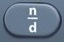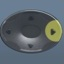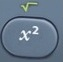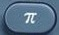# ToggleBasics
The toggle button enables switching between results:
• fraction ⇔ decimal
• square root ⇔ decimal
• π ⇔ decimalis the toggle command.

Question

What is$\bf\displaystyle\frac{3}{4}$ in decimal form?
0.75

Question
What is 0.75 in fraction form?$\bf\displaystyle\frac{3}{4}$

Input Display Commentblinker clears screen34$\bf\displaystyle\frac{3}{4}$$\bf\displaystyle\frac{3}{4}$0.75 Toggle$\bf\displaystyle\frac{3}{4}$ Toggle
###### Toggle

Question

What is √13 in decimal form?
3.605551275

Question
What is 3.605551275 in square root form?
13

Input Display Commentblinker clears screen13

13133.605551275 Toggle13 Toggle
###### Toggle

Question

What is 6 × π in decimal form?
18.84955592

Question
What is 18.84955592 in π form?

Input Display Commentblinker clears screen
6 ×6*π18.84955592 ToggleToggle
###### Toggle

Practice – Questions

1.  What is$\bf\displaystyle\frac{3}{8}$ in decimal form?

2.  What is 0.375 in fraction form?

3.  What is$\bf\displaystyle\frac{5}{6}$ in decimal form?

4.  What is 0.833333333 in fraction form?

5.  What is √11 in decimal form?

6.  What is 3.31662479 in square root form?

7.  What is 4 × √5 in decimal form?

8.  What is 8.94427191 in square root form?

9.  What is 3 × π in decimal form?

10.  What is 9.424777961 in π form?

1.  0.375

2.$\bf\displaystyle\frac{3}{8}$

3.  0.833333333

4.$\bf\displaystyle\frac{5}{6}$

5.  3.31662479

6.  √11

7.  8.94427191

8.  4√5

9.  9.424777961

10.  3π

## 2 thoughts on “Toggle”

1.Peg Harvey says:

My GED students THANK YOU!!

1.Scott Solomon says:

Peg,

Thank you for being there for your GED students.

Scott